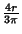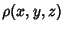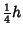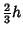## Centroid (Geometric)

The Center of Mass of a 2-D planar Lamina or a 3-D solid. The mass of a Lamina with surface density functionis(1)

The coordinates of the centroid (also called the Center of Gravity) are(2)(3)

The centroids of several common laminas along the nonsymmetrical axis are summarized in the following table.

 FigureParabolic SegmentSemicircleIn 3-D, the mass of a solid with density functionis(4)

and the coordinates of the center of mass are(5)(6)(7)

 FigureConeConical FrustumHemisphereParaboloidPyramidMcLean, W. G. and Nelson, E. W. First Moments and Centroids.'' Ch. 9 in Schaum's Outline of Theory and Problems of Engineering Mechanics: Statics and Dynamics, 4th ed. New York: McGraw-Hill, pp. 134-162, 1988.